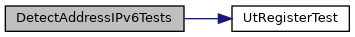suricata
This graph shows which files directly or indirectly include this file: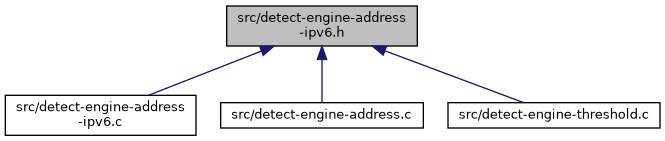Go to the source code of this file.

## Functions

Compares 2 ipv6 addresses and returns if the first address(a) is less than the second address(b) or not. More...

Compares 2 ipv6 addresses and returns if the first address(a) is greater than the second address(b) or not. More...

Compares 2 ipv6 addresses and returns if the addresses are equal or not. More...

Compares 2 ipv6 addresses and returns if the first address(a) is less than or equal to the second address(b) or not. More...

Compares 2 ipv6 addresses and returns if the first address(a) is greater than or equal to the second address(b) or not. More...

int AddressIPv6LeU32 (uint32_t *a, uint32_t *b)

int AddressIPv6LtU32 (uint32_t *a, uint32_t *b)

int AddressIPv6GtU32 (uint32_t *a, uint32_t *b)

int AddressIPv6EqU32 (uint32_t *a, uint32_t *b)

int AddressIPv6GeU32 (uint32_t *a, uint32_t *b)

Cuts and returns an address range, which is the complement of the address range that is supplied as the argument. More...

## Function Documentation

Compares 2 ipv6 addresses and returns if the addresses are equal or not.

Parameters
 a The first ipv6 address to be compared. b The second ipv6 address to be compared.
Return values
 1 If a == b. 0 Otherwise.

Definition at line 128 of file detect-engine-address-ipv6.c.

Here is the caller graph for this function:int AddressIPv6EqU32 ( uint32_t * a, uint32_t * b )

Definition at line 140 of file detect-engine-address-ipv6.c.

Here is the caller graph for this function: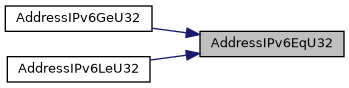Compares 2 ipv6 addresses and returns if the first address(a) is greater than or equal to the second address(b) or not.

Parameters
 a The first ipv6 address to be compared. b The second ipv6 address to be compared.
Return values
 1 If a >= b. 0 Otherwise, i.e. a < b.

Definition at line 194 of file detect-engine-address-ipv6.c.

Here is the call graph for this function:Here is the caller graph for this function:int AddressIPv6GeU32 ( uint32_t * a, uint32_t * b )

Definition at line 205 of file detect-engine-address-ipv6.c.

Here is the call graph for this function: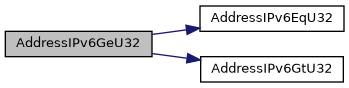Compares 2 ipv6 addresses and returns if the first address(a) is greater than the second address(b) or not.

Parameters
 a The first ipv6 address to be compared. b The second ipv6 address to be compared.
Return values
 1 If a > b. 0 Otherwise, i.e. a <= b.

Definition at line 90 of file detect-engine-address-ipv6.c.

References SCNtohl.

Here is the caller graph for this function:int AddressIPv6GtU32 ( uint32_t * a, uint32_t * b )

Definition at line 104 of file detect-engine-address-ipv6.c.

References SCNtohl.

Here is the caller graph for this function: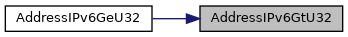Compares 2 ipv6 addresses and returns if the first address(a) is less than or equal to the second address(b) or not.

Parameters
 a The first ipv6 address to be compared. b The second ipv6 address to be compared.
Return values
 1 If a <= b. 0 Otherwise, i.e. a > b.

Definition at line 162 of file detect-engine-address-ipv6.c.

Here is the call graph for this function:Here is the caller graph for this function:int AddressIPv6LeU32 ( uint32_t * a, uint32_t * b )

Definition at line 173 of file detect-engine-address-ipv6.c.

Here is the call graph for this function: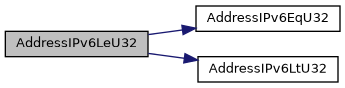Compares 2 ipv6 addresses and returns if the first address(a) is less than the second address(b) or not.

Parameters
 a The first ipv6 address to be compared. b The second ipv6 address to be compared.
Return values
 1 If a < b. 0 Otherwise, i.e. a >= b.

Definition at line 52 of file detect-engine-address-ipv6.c.

References SCNtohl.

Here is the caller graph for this function:int AddressIPv6LtU32 ( uint32_t * a, uint32_t * b )

Definition at line 66 of file detect-engine-address-ipv6.c.

References SCNtohl.

Here is the caller graph for this function: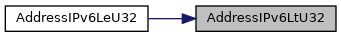Parameters
 a Pointer to the first address instance to be compared. b Pointer to the second address instance to be compared.
Return values

Definition at line 232 of file detect-engine-address-ipv6.c.

Here is the call graph for this function: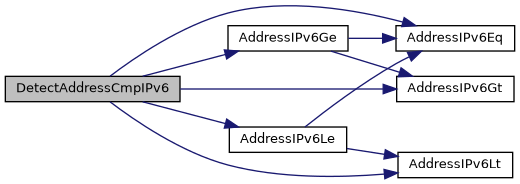Here is the caller graph for this function: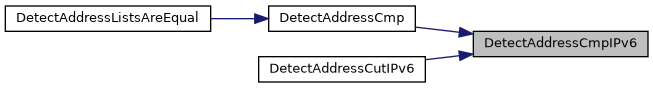Definition at line 359 of file detect-engine-address-ipv6.c.

Here is the call graph for this function: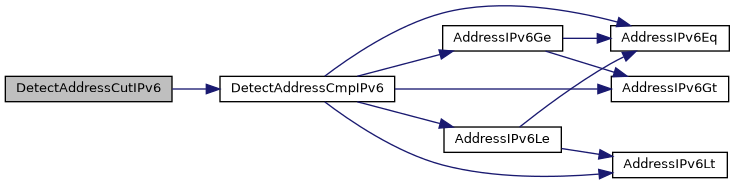Cuts and returns an address range, which is the complement of the address range that is supplied as the argument.

For example:

If a = ::-2000::, then a = 2000::1-FFFF:FFFF:FFFF:FFFF:FFFF:FFFF:FFFF:FFFF and b = NULL If a = 2000::1-FFFF:FFFF:FFFF:FFFF:FFFF:FFFF:FFFF:FFFF, then a = ::-2000:: and b = NULL If a = 2000::1-20FF::2, then a = ::-2000:: and b = 20FF::3-FFFF:FFFF:FFFF:FFFF:FFFF:FFFF:FFFF:FFFF

Parameters
 a Pointer to an address range (DetectAddress) instance whose complement has to be returned in a and b. b Pointer to DetectAddress pointer, that will be supplied back with a new DetectAddress instance, if the complement demands so.
Return values
 0 On success. -1 On failure.

Definition at line 710 of file detect-engine-address-ipv6.c.